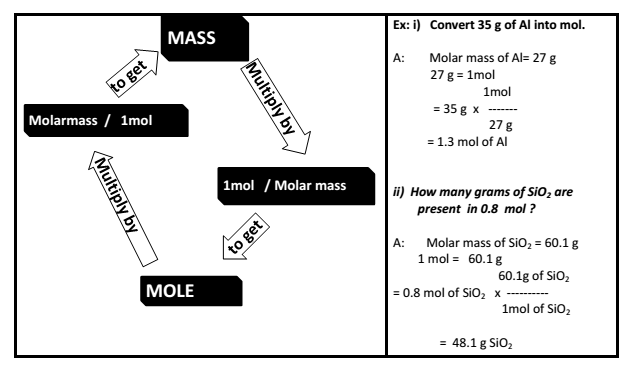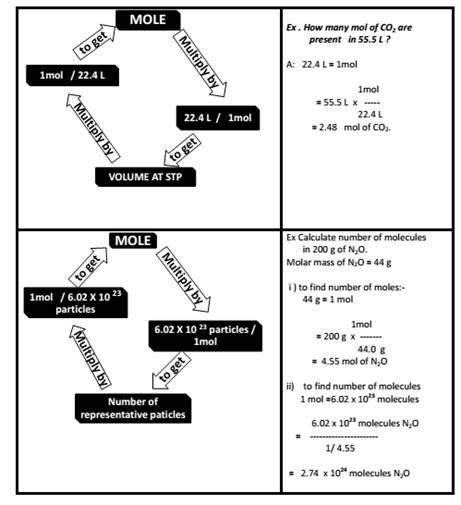### Atoms and Molecules - Revision Notes

CBSE Class 9 Science

Revision Notes
CHAPTER – 3

Atoms and Molecules

1. Laws of Chemical Combination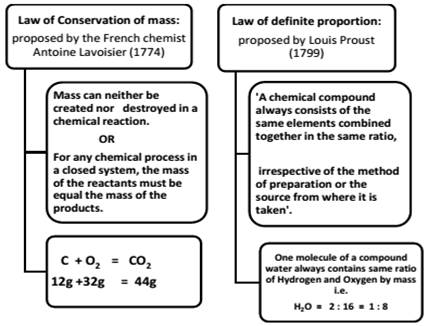Limitation of “Law of definite proportion”
This law does not hold good when the compound is obtained by using different isotopes of the combining elements.

2. John Daltons Atomic Theory
Using his theory, Dalton rationalized the various laws of chemical combination which were in existence at that time. However, he assumed that the simplest compound of two elements must be binary.

3. Atoms, Molecules, Ions & Chemical Formula
Atom
An atom is the smallest particle of an element which can take part in a chemical reaction. It may or may not exist freely.

$↓$

Each atom of an element shows all the properties of the element.

Molecule
The smallest particle of matter (element or compound) which can exist in a free state.

$↓$

The properties of a substance are the properties of its molecules.

• MOLECULES OF ELEMENT : The molecules of an element are constituted by the same type of atoms.
• MOLECULES OF COMPOUND: Atoms of different elements join together in definite proportions to form molecules of compounds. (hetero atomic molecules)
• ATOMICITY: The number of atoms contained in a molecule of a substance (element or compound) is called its atomic
 Element Formula Atomicity Ozone O3 3 Phosphorus P4 4 Sulphur S8 8 Oxygen O2 2
• Based upon atomicity molecules can be classified as follows.
• Monoatomic molecules: Noble gases helium, neon and argon exist as He Ne and Ar respectively.

Diatomic molecules${H}_{2},\phantom{\rule{thickmathspace}{0ex}}{O}_{2},\phantom{\rule{thickmathspace}{0ex}}{N}_{2},\phantom{\rule{thickmathspace}{0ex}}C{l}_{2},\phantom{\rule{thickmathspace}{0ex}}CO,\phantom{\rule{thickmathspace}{0ex}}HCl.$
Triatomic molecules${O}_{3},\phantom{\rule{thickmathspace}{0ex}}C{O}_{2},\phantom{\rule{thickmathspace}{0ex}}N{O}_{2}$

SYMBOLS

• The abbreviation used to represent an element is generally the first letter in capital of the English name of element.
Oxygen $\to$ O Nitrogen $\to$ N
• When the names of two or more elements begin with the same initial letter, the initial letter followed by the letter appearing later in the name is used to symbolize the element
Barium $\to$ Ba Bismuth $\to$ Bi

Symbols of some elements are derived from their Latin names

 ELEMENT LATIN NAME SYMBOL Sodium Natrium Na Copper Cuprum Cu Potassium Kalium K Iron Ferrum Fe Mercury Hydragyrum Hg Tungsten Wolfram W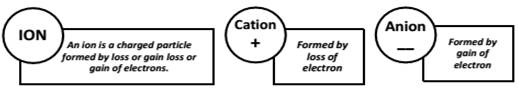Polyatomic Ion : A group of atoms carrying a charge is as polyatomic ion.
Eg. $\begin{array}{c}N{{H}_{4}}^{+}-Ammoniumlon:C{{O}_{3}}^{2-}-Carbonate\phantom{\rule{thickmathspace}{0ex}}ion\end{array}$

Valency : The number of electrons which an atom can lose , gain or share to form a bond.

OR

It is the combining capacity of an atom of the element.

• Chemical Formula : A chemical formula is a short method of representing chemical elements and compounds.

Writing a Chemical Formula -CRISS-CROSS rule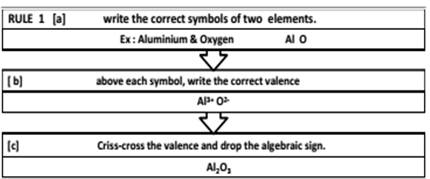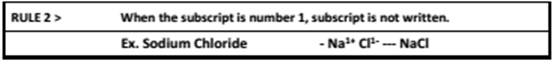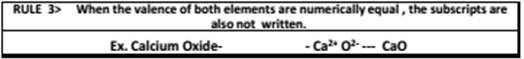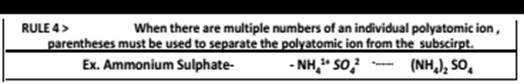RULES > All subscripts must be reduced to lowest term (except for molecular or
Examples

 CATION ANION FORMULA NAME Al3+$A{l}^{3+}$ SO42−$S{{O}_{4}}^{2-}$ Al2(SO4)3$A{l}_{2}\left(S{O}_{4}{\right)}_{3}$ Aluminium sulphate Ca HCO3−$HC{{O}_{3}}^{-}$ Ca(HCO3)2$Ca\left(HC{O}_{3}{\right)}_{2}$ Calcium bicarbonate NH4+$N{{H}_{4}}^{+}$ Cl−$C{l}^{-}$ NH4Cl$N{H}_{4}Cl$ Ammonium chloride Na+$N{a}^{+}$ CO32−$C{{O}_{3}}^{2-}$ HCO3−$HC{{O}_{3}}^{-}$ Sodium carbonate Mg2+$M{g}^{2+}$ OH−$O{H}^{-}$ Mg(OH)2$Mg\left(OH{\right)}_{2}$ Magnesium hydroxide Na+$N{a}^{+}$ PO43−$P{{O}_{4}}^{3-}$ Na3PO4$N{a}_{3}P{O}_{4}$ Sodium phosphate

4. Mole Concept

The mole (mol) is the amount of a substance that contains as many elementary entities as there are atoms in exactly 12.00 grams of ${}^{12}C$

The Avogadro constant is named after the early nineteenth century Italian scientist Amedeo Avogadro.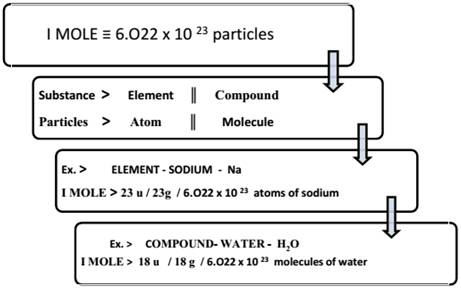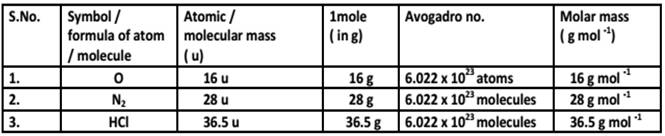GRAM MOLECULAR MASS
Gram molecular mass is the mass in grams of one mole of a molecular substance.
Ex: The molecular mass of ${N}_{2}$ is 28, so the gram molecular mass of ${N}_{2}$ is 28 g.

ATOMIC MASS UNIT
An atomic mass unit or amu is one twelfth of the mass of an unbound atom of carbon-12. It is a unit of mass used to express atomic masses and molecular masses.
Also Known As: Unified Atomic Mass Unit (u).

MOLECULAR MASS : A number equal to the sum of the atomic masses of the atoms in a molecule. The molecular mass gives the mass of a molecule relative to that of the 12 C atom, which is taken to have a mass of 12.
Examples: The molecular mass of ${C}_{2}{H}_{6}$ is approximately 30 or . Therefore the molecule is about 2.5 times as heavy as the 12C atom or about the same mass as the NO atom with a molecular mass of 30 or (14 +16).

5. Molar Mass & Avogadro Constant